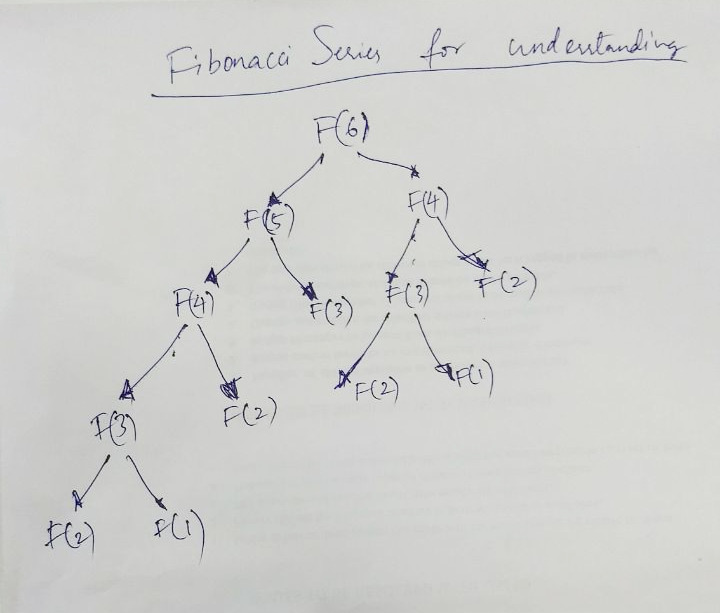# Question & Answer: A computer implementation of a number of problems covering the area:…..

ALGORITHMS ANALYSIS

A computer implementation of a number of problems covering the area:

Don't use plagiarized sources. Get Your Custom Essay on
Question & Answer: A computer implementation of a number of problems covering the area:…..
GET AN ESSAY WRITTEN FOR YOU FROM AS LOW AS \$13/PAGE

1. Divide and conquer

2. Dynamic programming and

3. Greedy algorithms

The student should also submit evidence that the program performs the required tasks correctly. And demonstrate a good grasp of the factors involved in the design of efficient algorithms.

You should submit a report with following included:

objective of the report

propsed soloution (flowchart)

Sample screen display (program run)

//Sample programming example of Implementing binary search using Divide and Conquer

//Explanation

A binary search or half-interval search algorithm finds the position of a specified value (the input “key”) within a sorted array.In each step, the algorithm compares the input key value with the key value of the middle element of the array. If the keys match, then a matching element has been found so its index, or position, is returned. Otherwise, if the sought key is less than the middle element’s key, then the algorithm repeats its action on the sub-array to the left of the middle element or, if the input key is greater, on the sub-array to the right. If the remaining array to be searched is reduced to zero, then the key cannot be found in the array and a special “Not found” indication is returned.

public class MyBinarySearch {

public int binarySearch(int[] inputArr, int key) {

int start = 0;

int end = inputArr.length – 1;

while (start <= end) {

int mid = (start + end) / 2;

if (key == inputArr[mid]) {

return mid;

}

if (key < inputArr[mid]) {

end = mid – 1;

} else {

start = mid + 1;

}

}

return -1;

}

public static void main(String[] args) {

MyBinarySearch mbs = new MyBinarySearch();

int[] arr = {2, 4, 6, 8, 10, 12, 14, 16};

System.out.println(“Key 14’s position: “+mbs.binarySearch(arr, 14));

int[] arr1 = {6,34,78,123,432,900};

System.out.println(“Key 432’s position: “+mbs.binarySearch(arr1, 432));

}

}

 Output: ```Key 14's position: 6 Key 432's position: 4 ``` Dynamic Programming is nothing but an simple recursion. simple example will illustrate below The classic example to explain dynamic programming is the fibonacci computation The definition of the fibonacci number of a number is clearly a recursive one: F(n) = F(n-1) + F(n-2) and F(1) = F(2) = 1 This means that the sequence of the first 10 fibonacci numbers would go: 1, 1, 2, 3, 5, 8, 13, 21, 34, 55 You might also find it defined as: F(0) = F(1) = 1 And so the sequence would be: 0,1, 1, 2, 3, 5, 8, 13, 21, 34, 55 //example public static long fibonacci(int n) { if (n < 3) return 1; return fibonacci(n-2) + fibonacci(n-1); } Greedy is an algorithmic paradigm that builds up a solution piece by piece, always choosing the next piece that offers the most obvious and immediate benefit. Greedy algorithms are used for optimization problems. An optimization problem can be solved using Greedy if the problem has the following property: At every step, we can make a choice that looks best at the moment, and we get the optimal solution of the complete problem. There are standard algorithm that are greedy algorithm such as krushkal mimimum spanning tree,prim minimum spanning tree, dijkshtra shortest p[ath,huffman coding ```Example 1 : Consider the following 3 activities sorted by by finish time. start[] = {10, 12, 20}; finish[] = {20, 25, 30}; A person can perform at most two activities. The maximum set of activities that can be executed is {0, 2} [ These are indexes in start[] and finish[] ] ``` / The following implementation assumes that the activities // are already sorted according to their finish time import java.util.*; import java.lang.*; import java.io.*; class ActivitySelection { // Prints a maximum set of activities that can be done by a single // person, one at a time. // n   –> Total number of activities // s[] –> An array that contains start time of all activities // f[] –> An array that contains finish time of all activities public static void printMaxActivities(int s[], int f[], int n) { int i, j;   System.out.print(“Following activities are selected : n”);   // The first activity always gets selected i = 0; System.out.print(i+” “);   // Consider rest of the activities for (j = 1; j < n; j++) { // If this activity has start time greater than or // equal to the finish time of previously selected // activity, then select it if (s[j] >= f[i]) { System.out.print(j+” “); i = j; } } }   // driver program to test above function public static void main(String[] args) { int s[] = {1, 3, 0, 5, 8, 5}; int f[] = {2, 4, 6, 7, 9, 9}; int n = s.length;   printMaxActivities(s, f, n); }   } ```Following activities are selected 0 1 3 4 ```